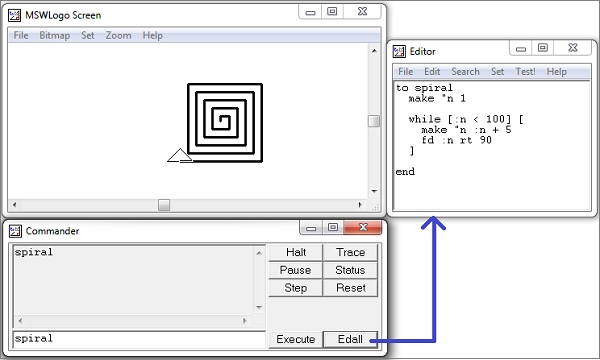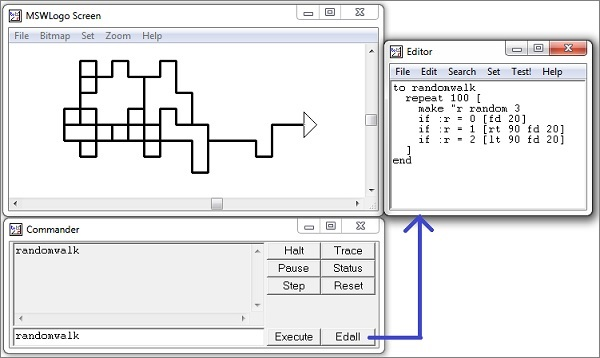# Logo - Decision-Making

Decision-making and variables go together. A program needs to be able to change course depending on the situation. Here, for example, is a framework for drawing a spiral. It has a loop, a variation on the repetition shown earlier and the body of the loop is for us to fill in.

```to spiral
make "n 1
while [:n < 100] [
make "n :n + 5
fd :n rt 90
]
end
```

The above code shows several new features of the syntax of the MSW Logo. We set a variable to a new value by keying-in ‘make’, then the variable's name is preceded by a double quote " rather than a colon ‘:’ as shown below.

```make "n 1
```

We use a variable, though, with a colon ‘:’ in front of its name.

```while [:n < 100]
```

The code bracketed after the ‘while [condition]’ is executed, while the condition is true. When it is no longer true, because (in this case) the value of ‘:n’ grows greater than 100, the code following the bracket is executed.

Following screenshot shows the execution and output of the above code.Now, we shall discuss the use of ‘if statements’, which have a code that will be executed only when a given condition is true.

It also shows a built-in Logo that generates random numbers. The statement random 3 generates any number 0 or 1 or 2 arbitrarily in a random sequence. The procedure then decides which way to go "at random". The generated random number will be kept in ‘r’ and later depending upon the value of the variable ‘r’ one of the if-statements will get executed, which will satisfy the condition. Thus if the −

• Value of ‘r’ is 0, then [fd 20] will be executed.
• Value of ‘r’ is 1, then [rt 90 fd 20] will be executed.
• Value of ‘r’ is 2, then [lt 90 fd 20] will be executed.

Following screenshot shows the execution and output of the above discussion.